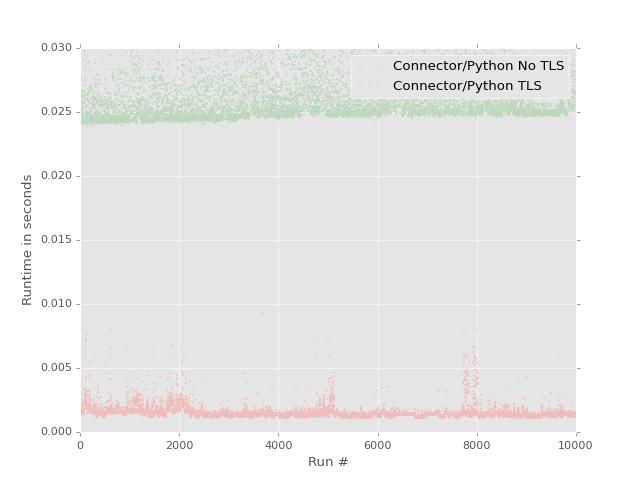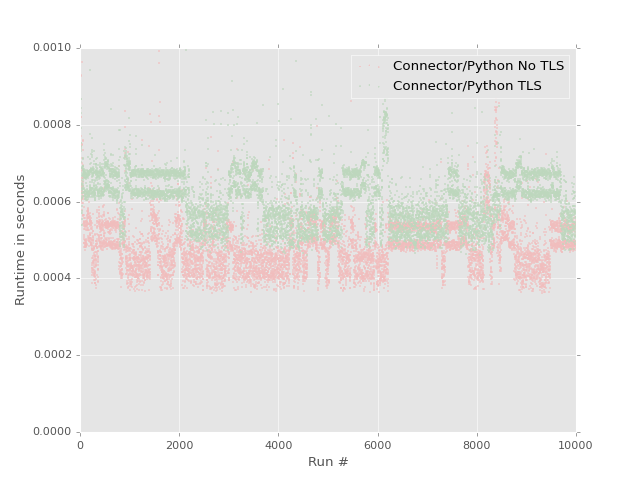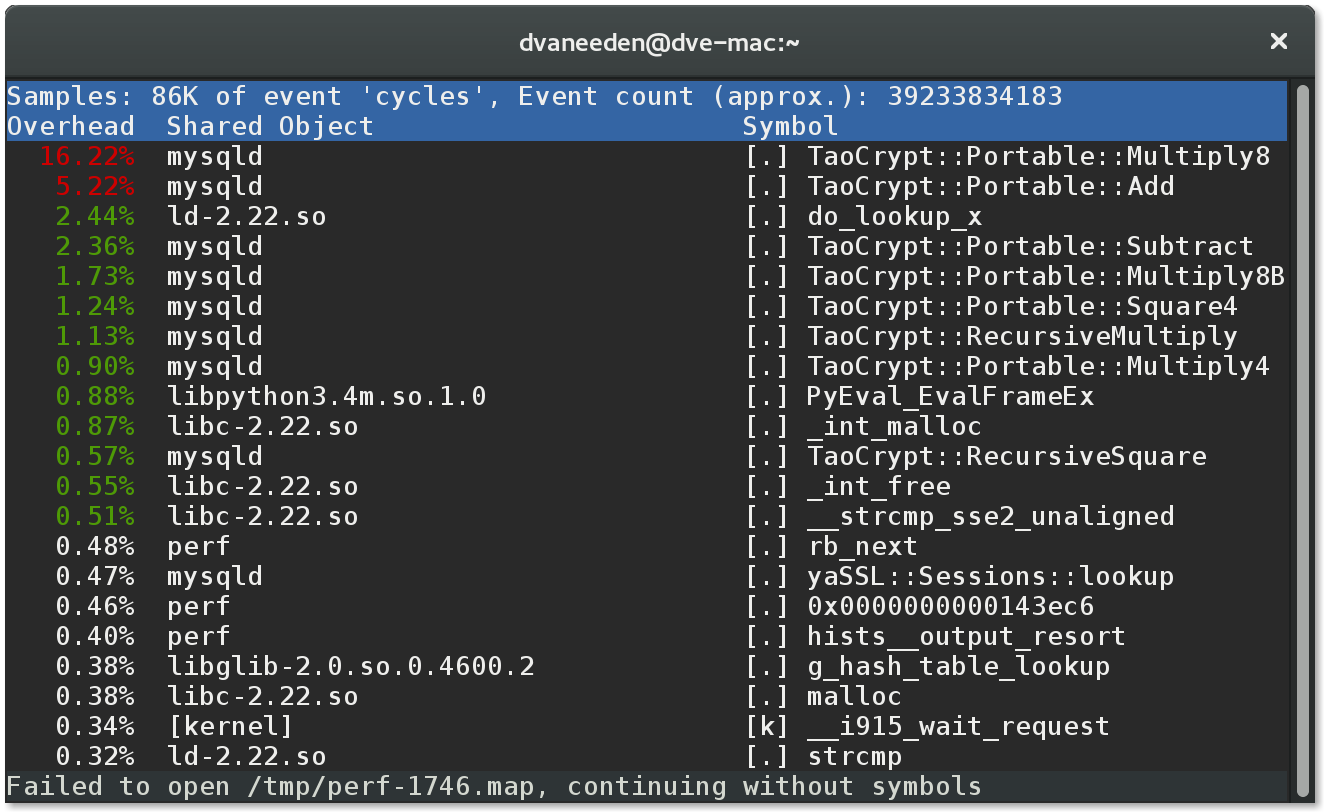After the test I did with the C Extension of MySQL Connector/Python I was curious how costly using Transport Layer Security (TLS) is.

Note: TLS is the successor of Secure Sockets Layer (SSL). None of the current MySQL versions support SSL at all, but all variable names still use SSL.

In :
import random
import gzip
import time

import pandas as pd
import matplotlib.pyplot as plt
import requests
import mysql.connector

print('Using MySQL Connector/Python {version}'.format(version=mysql.connector.__version__))

Using MySQL Connector/Python 2.1.3

In :
worlddb_url = 'https://downloads.mysql.com/docs/world.sql.gz'
worlddb_req = requests.get(worlddb_url)
if worlddb_req.status_code == 200:
worldsql = gzip.decompress(worlddb_req.content).decode('iso-8859-15')


I've used mysql_ssl_rsa_setup to enable TLS on MySQL 5.7.10 running in MySQL Sandbox.

In :
config = {
'host': '127.0.0.1',
'port': 5710,
'user': 'msandbox',
'passwd': 'msandbox',
}
tls_ca_file = '/home/dvaneeden/sandboxes/msb_5_7_10/data/ca.pem'

In :
c1 = mysql.connector.connect(ssl_ca=tls_ca_file, **config)
cur1 = c1.cursor()
cur1.execute('DROP SCHEMA IF EXISTS world')
cur1.execute('CREATE SCHEMA world DEFAULT CHARACTER SET latin1')
cur1.execute('USE world')
result = [x for x in cur1.execute(worldsql, multi=True)]
cur1.close()
c1.close()

In :
c1 = mysql.connector.connect(ssl_ca=tls_ca_file, **config)
cur1 = c1.cursor()
cur1.execute("SHOW SESSION STATUS LIKE 'SSL%'")
tls_conn = {x: x for x in cur1 if x in ['Ssl_version','Ssl_cipher']}
print("Using protocol %s and cipher %s" % (tls_conn['Ssl_version'], tls_conn['Ssl_cipher']))
c1.close()

Using protocol TLSv1 and cipher DHE-RSA-AES256-SHA

In :
config['db'] = 'world'

In :
perfdata = pd.DataFrame(columns=['connpy','connpy_tls'], index=range(10000))

In :
for it in range(10000):
city_id = random.randint(1,8000)
start = time.perf_counter()
c1 = mysql.connector.connect(ssl_ca=tls_ca_file, **config)
cur1 = c1.cursor()
cur1.execute("SELECT * FROM City WHERE ID=%s", (city_id,))
cur1.fetchone()
c1.close()
perfdata.ix[it]['connpy_tls'] = time.perf_counter() - start

In :
for it in range(10000):
city_id = random.randint(1,8000)
start = time.perf_counter()
c1 = mysql.connector.connect(**config)
cur1 = c1.cursor()
cur1.execute("SELECT * FROM City WHERE ID=%s", (city_id,))
cur1.fetchone()
c1.close()
perfdata.ix[it]['connpy'] = time.perf_counter() - start


Now let's have a look to what our data looks like

In :
perfdata.head()

Out:
connpy connpy_tls
0 0.00187417 0.0331963
1 0.00171487 0.0277591
2 0.0015613 0.0251479
3 0.00134424 0.024894
4 0.00149395 0.028625

Now let's plot that

In :
%matplotlib notebook
plt.style.use('ggplot')
plt.scatter(perfdata.index, perfdata.connpy, s=1, c='r',
label='Connector/Python No TLS')
plt.scatter(perfdata.index, perfdata.connpy_tls, s=1, c='g',
label='Connector/Python TLS')
plt.ylim(ymin=0, ymax=0.03)
plt.xlim(xmin=0, xmax=10000)
plt.xlabel('Run #')
plt.ylabel('Runtime in seconds')
plt.legend()Out:
<matplotlib.legend.Legend at 0x7f1c05886f98>

The performance of MySQL Connector/Python 2.1 with TLS looks much slower than without TLS.

But we create a new connection for each query. This is the worst-case senario.

What would happen if we would use a persistend connection?

In :
perfdata2 = pd.DataFrame(columns=['p_connpy','p_connpy_tls'], index=range(10000))

In :
c1 = mysql.connector.connect(**config)
for it in range(10000):
city_id = random.randint(1,8000)
start = time.perf_counter()
cur1 = c1.cursor()
cur1.execute("SELECT * FROM City WHERE ID=%s", (city_id,))
cur1.fetchone()
perfdata2.ix[it]['p_connpy'] = time.perf_counter() - start
c1.close()

In :
c1 = mysql.connector.connect(ssl_ca=tls_ca_file, **config)
for it in range(10000):
city_id = random.randint(1,8000)
start = time.perf_counter()
cur1 = c1.cursor()
cur1.execute("SELECT * FROM City WHERE ID=%s", (city_id,))
cur1.fetchone()
perfdata2.ix[it]['p_connpy_tls'] = time.perf_counter() - start
c1.close()

In :
%matplotlib notebook
plt.style.use('ggplot')
plt.scatter(perfdata2.index, perfdata2.p_connpy, s=1, c='r',
label='Connector/Python No TLS')
plt.scatter(perfdata2.index, perfdata2.p_connpy_tls, s=1, c='g',
label='Connector/Python TLS')
plt.ylim(ymin=0, ymax=0.001)
plt.xlim(xmin=0, xmax=10000)
plt.xlabel('Run #')
plt.ylabel('Runtime in seconds')
plt.legend()Out:
<matplotlib.legend.Legend at 0x7f1c0716ebe0>

This shows that not reconnecting for each and every query can make a huge difference when using TLS.

The difference doesn't have to be as big. Using TLS Tickets could reduce the reconnect cost. See also MySQL Bug #76921

I did this test also with the C Extension enabled, and the results were similar. But testing was a bit more complicated as there is no option to force TLS to be disabled. See also MySQL Bug #79825.

The perf top output below was taken when running the test with TLS and reconnects for every statement.

In :
from IPython.display import Image
Image(filename="perf_top_mysql_ssl.png")

Out: